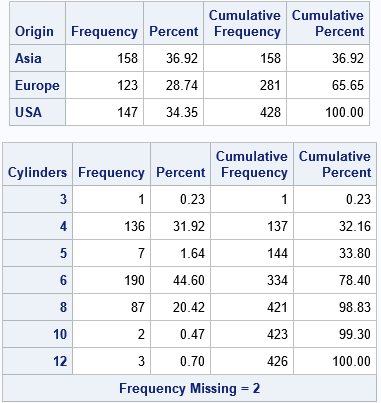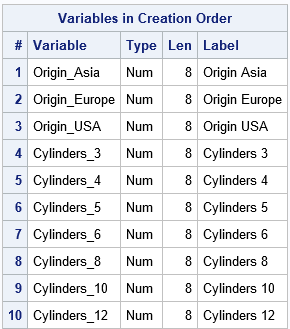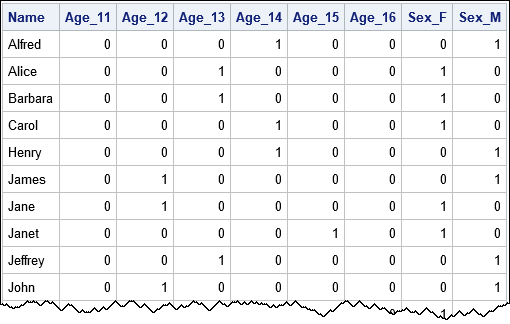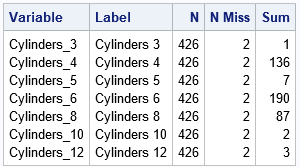On discussion forums, many SAS programmers ask about the best way to generate dummy variables for categorical variables. Well-meaning responders offer all sorts of advice, including writing your own DATA step program, sometimes mixed with macro programming. This article shows that the simplest and easiest way to generate dummy variables in SAS is to use PROC GLMSELECT. It is not necessary to write a SAS program to generate dummy variables. This article shows an example of generating dummy variables that have meaningful names, which are based on the name of the original variable and the categories (levels) of the variable.

A dummy variable is a binary indicator variable. Given a categorical variable, X, that has k levels, you can generate k dummy variables. The j_th dummmy variable indicates the presence (1) or absence (0) of the j_th category.

### Why GLMSELECT is the best way to generate dummy variables

I usually avoid saying "this is the best way" to do something in SAS. But if you are facing an impending deadline, you are probably more interested in solving your problem and less interested in comparing five different ways to solve it. So let's cut to the chase: If you want to generate dummy variables in SAS, use PROC GLMSELECT.

Why do I say that? Because PROC GLMSELECT has the following features that make it easy to use and flexible:

• The syntax of PROC GLMSELECT is straightforward and easy to understand.
• The dummy variables that PROC GLMSELECT creates have meaningful names. For example, if the name of the categorical variable is X and it has values 'A', 'B', and 'C', then the names of the dummy variables are X_A, X_B, and X_C.
• PROC GLMSELECT creates a macro variable named _GLSMOD that contains the names of the dummy variables.
• When you write the dummy variables to a SAS data set, you can include the original variables or not.
• By default, PROC GLMSELECT uses the GLM parameterization of CLASS variables. This is what you need to generate dummy variables. But the same procedure also enables you to generate design matrices that use different parameterizations, that contain interaction effects, that contain spline bases, and more.

The only drawback to using PROC GLMSELECT is that it requires a response variable to put on the MODEL statement. But that is easily addressed.

### How to generate dummy variables

Let's show an example of generating dummy variables. I will use two categorical variables in the Sashelp.Cars data: Origin and Cylinders. First, let's look at the data. As the output from PROC FREQ shows, the Origin variable has three levels ('Asia', 'Europe', and 'USA') and the Cylinders variable has seven valid levels and also contains two missing values.

```%let DSIn = Sashelp.Cars; /* name of input data set */ %let VarList = Origin Cylinders; /* name of categorical variables */   proc freq data=&DSIn; tables &VarList; run;```In order to use PROC GLMSELECT, you need a numeric response variable. PROC GLMSELECT does not care what the response variable is, but it must exist. The simplest thing to do is to create a "fake" response variable by using a DATA step view. To generate the dummy variables, put the names of the categorical variables on the CLASS and MODEL statements. You can use the OUTDESIGN= option to write the dummy variables (and, optionally, the original variables) to a SAS data set. The following statements generate dummy variables for the Origin and Cylinders variables:

```/* An easy way to generate dummy variables is to use PROC GLMSELECT */ /* 1. add a fake response variable */ data AddFakeY / view=AddFakeY; set &DSIn; _Y = 0; run;   /* 2. Create the dummy variables as a GLM design matrix. Include the original variables, if desired */ proc glmselect data=AddFakeY NOPRINT outdesign(addinputvars)=Want(drop=_Y); class &VarList; /* list the categorical variables here */ model _Y = &VarList / noint selection=none; run;```

The dummy variables are contained in the WANT data set. As mentioned, the GLMSELECT procedure creates a macro variable (_GLSMOD) that contains the names of the dummy variables. You can use this macro variable in procedures and in the DATA step. For example, you can use it to look at the names and labels for the dummy variables:

```/* show the names of the dummy variables */ proc contents varnum data=Want(keep=&_GLSMOD); ods select Position; run;```Notice that the names of the dummy variables are very understandable. The three levels of the Origin variable are 'Asia', 'Europe', and 'USA, so the dummy variables are named Origin_Asia, Origin_Europe, and Origin_USA. The dummy variables for the seven valid levels of the Cylinders variable are named Cylinders_N, where N is a valid level.

### A macro to generate dummy variables

It is easy to encapsulate the two steps into a SAS macro to make it easier to generate dummy variables. The following statements define the %DummyVars macro, which takes three arguments:

1. DSIn is the name of the input data set, which contains the categorical variables.
2. VarList is a space-separated list of the names of the categorical variables. Dummy variables will be created for each variable that you specify.
3. DSOut is the name of the output data set, which contains the dummy variables.
```/* define a macro to create dummy variables */ %macro DummyVars(DSIn, /* the name of the input data set */ VarList, /* the names of the categorical variables */ DSOut); /* the name of the output data set */ /* 1. add a fake response variable */ data AddFakeY / view=AddFakeY; set &DSIn; _Y = 0; /* add a fake response variable */ run; /* 2. Create the design matrix. Include the original variables, if desired */ proc glmselect data=AddFakeY NOPRINT outdesign(addinputvars)=&DSOut(drop=_Y); class &VarList; model _Y = &VarList / noint selection=none; run; %mend;   /* test macro on the Age and Sex variables of the Sashelp.Class data */ %DummyVars(Sashelp.Class, Age Sex, ClassDummy);```

When you run the macro, it writes the dummy variables to the ClassDummy data set. It also creates a macro variable (_GLSMOD) that contains the name of the dummy variables. You can use the macro to analyze or print the dummy variables, as follows:

```/* _GLSMOD is a macro variable that contains the names of the dummy variables */ proc print data=ClassDummy noobs; var Name &_GLSMod; run;```The dummy variables tell you that Alfred is a 14-year-old male, Alice is a 13-year-old female, and so forth.

### What happens if a categorical variable contains a missing value?

If a categorical variable contains a missing value, so do all dummy variables that are generated from that variable. For example, we saw earlier that the Cylinders variable for the Sashelp.Cars data has two missing values. You can use PROC MEANS to show that the dummy variables (named Cylinders_N) also have two missing values. Because the dummy variables are binary variables, the sum of each dummy variable matches the number of levels. Compare the SUM column in the PROC MEANS output with the earlier output from PROC FREQ:

```/* A missing value in Cylinders results in a missing value for each dummy variable that is generated from Cylinders */ proc means data=Want N NMiss Sum ndec=0; vars Cylinders_:; run;```### Summary

In most analyses, it is unnecessary to generate dummy variables. Most SAS procedures support the CLASS statement, which enables you to use categorical variables directly in statistical analyses. However, if you do need to generate dummy variables, there is an easy way to do it: Use PROC GLMSELECT or use the %DummyVars macro in this article. The result is a SAS data set that contains the dummy variables and a macro variable (_GLSMOD) that contains the names of the dummy variables.

Here are links to previous articles about dummy variables and creating design matrices in SAS.

ShareDistinguished Researcher in Computational Statistics

Rick Wicklin, PhD, is a distinguished researcher in computational statistics at SAS and is a principal developer of PROC IML and SAS/IML Studio. His areas of expertise include computational statistics, simulation, statistical graphics, and modern methods in statistical data analysis. Rick is author of the books Statistical Programming with SAS/IML Software and Simulating Data with SAS.

1.2.•